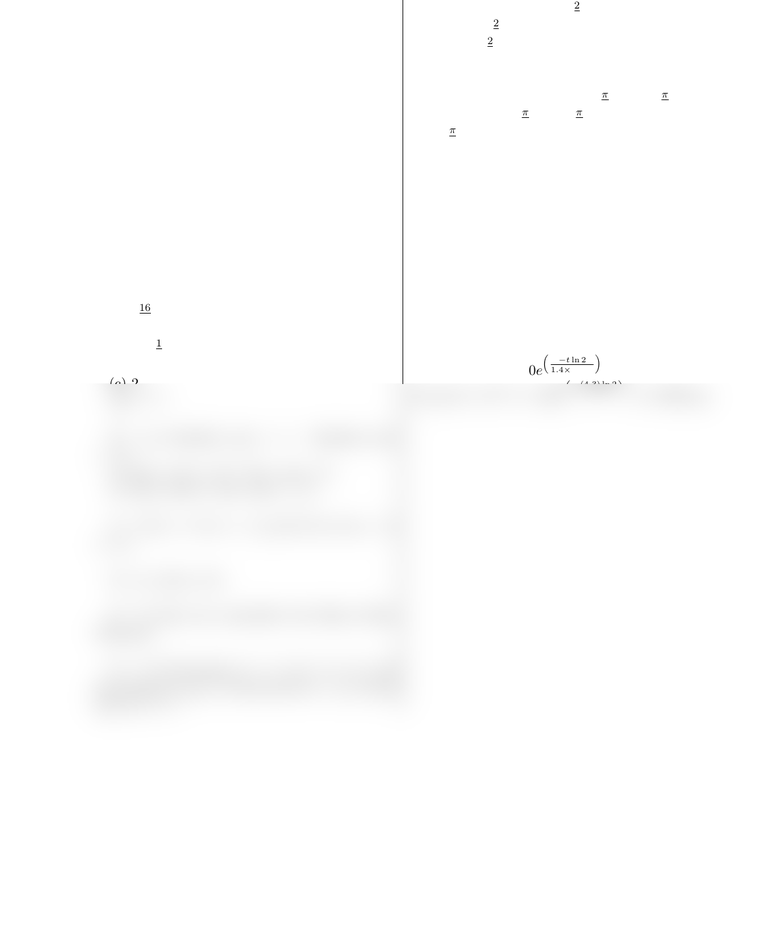Study Guides (380,000)
US (220,000)
MATH (20)
All (8)
Midterm

# MATH 165 Ball State Exam3Review165 SolutionExam

Department
Mathematical Sciences
Course Code
MATH 165
Professor
All
Study Guide
Midterm

This preview shows half of the first page. to view the full 1 pages of the document.Maths 165
Review Sheet 3
1. 9.99
2. 9.99
4. y=xis a slant asymptote (as x→ −∞)
5. When x= 1,000/3, then P(x) = ...
6. 2.68269579
7. 1.01395761
8. 16
5
9. (a) -1
2
(b) 2
(c) 2
(d) e1/2
10. (a) Absolute max = 7. Absolute min
=9.
(b) Abs. max = 4e2. Abs. min = 0.
(c) Abs. max 0. Abs. min = 6.
11. f(x) = 0 at x=1
3and f(x) d.n.e. at
x= 0.
12. 4 f(4) 16.
13. It does not contradict the Mean Value
Theorem!
14. (a) Increasing on (−∞,1
3)(1,) and
decreasing on (1
3,1). Local max at x=1
3. Local
min at x= 1.
(b) Increasing on (2,2
5) and decreasing on
(−∞,2) (2
5,). Local min at x=2, Local
max at x=2
5.
(c) Decreasing on (−∞,0) and increasing on
(0,). Local min at x= 0.
(d) Increasing on (π, π
2)(0,π
2) and
decreasing on (π
2,0) (π
2, π). Local max at
x=±π
2and local min at x= 0.
15. It is always concave up. No inﬂection
points!
16. About 2.33 hours.
17. 2.
18. f(x) = 3ex5 sin(x) + 4x2.
19. (a) v(t) = 80 40tand x(t) = 80t20t2.
(b) No.
20. (a) p(t) = 100e³tln 2
1.4×104´
(b) p(4.3×104) = 100e³(4.3) ln 2
1.4´11.896 mg
###### You're Reading a Preview

Unlock to view full version